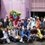# Triangle Area with Matrices

Hello,I'm Leonardo. I want to ask something that make me curious until now. At school, we have already learned that triangle area can derived from many things, like a standard formula (half times base times height), sine formula, cosine formula, and Heron's Formula. When I try to find another way to calculate the area, I just found that we can use matrices to find the area. But, I still don't understand how can matrices help us to find the area of triangle? For example, if we want to find the area of triangle with vertices A(0,9), B(3,7), and C(2,4), what is the shortest way to find this area without draw it in the Cartesius coordinate? Is matrices formula for triangle area have any criteria? Can we use it to find another polygon area? ThanksNote by Leonardo Chandra
6 years, 2 months ago

MarkdownAppears as
*italics* or _italics_ italics
**bold** or __bold__ bold
- bulleted- list
• bulleted
• list
1. numbered2. list
1. numbered
2. list
Note: you must add a full line of space before and after lists for them to show up correctly
paragraph 1paragraph 2

paragraph 1

paragraph 2

[example link](https://brilliant.org)example link
> This is a quote
This is a quote
    # I indented these lines
# 4 spaces, and now they show
# up as a code block.

print "hello world"
# I indented these lines
# 4 spaces, and now they show
# up as a code block.

print "hello world"
MathAppears as
Remember to wrap math in $ ... $ or $ ... $ to ensure proper formatting.
2 \times 3 $2 \times 3$
2^{34} $2^{34}$
a_{i-1} $a_{i-1}$
\frac{2}{3} $\frac{2}{3}$
\sqrt{2} $\sqrt{2}$
\sum_{i=1}^3 $\sum_{i=1}^3$
\sin \theta $\sin \theta$
\boxed{123} $\boxed{123}$

## Comments

Sort by:

Top Newest

Here is a proof of the formula for the area of a triangle with determinants. It's not exactly matrices that help with finding the area, it's the related topic of determinants. For the triangle with those points above you would have to solve the following determinant: $\left| \begin{array}{ccc} 0 & 9 & 1 \\ 3 & 7 & 1 \\ 2 & 4 & 1 \end{array} \right|=11$ Then you must take the absolute value of half of it to obtain your area. The determinant is set up with the x's in the first column, the y's in the second, and 1's in the third. There is no prior criteria for this method, besides being the most useful when you have the Cartesian coordinates of the vertices. It seems like this method only works for triangles in two dimensions, for increasing the number of points makes the determinant not-square, and there is no such thing as a not-square determinant. However, it seems the method can be extended into objects with n+1 edges in n dimensions.

- 6 years, 2 months ago

Log in to reply

Indeed. This is known as the shoelace method, in reference to how you multiply cross terms. Note that there is a factor of $\frac{1}{2}$ in front. It generalizes to finding the (signed) area of $n$ points in the plane, as mentioned by Hero.

It does generalize to objects with $n+1$ vertices in $n$ dimensions, but you're not finding the surface area, but rather the 'volume'. (There is a corresponding factor too, which I believe would be $\frac{1}{n!}$ .) Recall that in 2-D, the volume of an object is known as the area. As an example of the generalization, in 1-D, the volume of an object is the length of the line segment, and the volume (i.e. length) between the points $a$ and $b$ is $a-b$, which corresponds the the determinant of the matrix

$\begin{pmatrix} a & 1 \\ b & 1 \\ \end{pmatrix}$.

Staff - 6 years, 2 months ago

Log in to reply

Actually, I just realized my error in extending it into n dimensions. It should be n+1 vertices, not edges. Secondly, the shoelace formula is facinating. Thanks for sharing.

- 6 years, 2 months ago

Log in to reply

The generalization for a triangle in $\mathbb{R}^3$ is given by formula (18) on the following page: http://mathworld.wolfram.com/TriangleArea.html

- 6 years, 2 months ago

Log in to reply

Interesting. Here is the hyperlink (for others' ease).

- 6 years, 2 months ago

Log in to reply

I also just noticed that you could triangulate (cut into triangles) other polygons and apply this process over and over again. I conjecture that this will reduce to the Shoelace method that Calvin has posted above.

- 6 years, 2 months ago

Log in to reply

shoulnt it be 11/2? as the area is half the determinant

- 6 years, 2 months ago

Log in to reply

The area is 11/2. I never said that 11 was the area of the triangle.

- 6 years, 2 months ago

Log in to reply

U can do the the dot product of AB And AC then divide it by two and take its absolute value.

- 6 years, 2 months ago

Log in to reply

×

Problem Loading...

Note Loading...

Set Loading...